# MP Board Class 12th Physics Important Questions Chapter 9 Ray Optics and Optical Instruments

## MP Board Class 12th Physics Important Questions Chapter 9 Ray Optics and Optical Instruments

### Ray Optics and Optical Instruments Important Questions

Ray Optics and Optical Instruments Objective Type Questions

Question 1.
Choose the correct answer of the following:

Question 1.
The virtual and smaller image can be obtained by :
(a) Plane mirror
(b) Convex mirror
(c) Concave mirror
(d) None of these
(b) Convex mirror

Question 2.
Keeping the incident ray unchanged if the mirror is rotated by 30° angle then the reflected ray will be deviated through :
(a) 30°
(b) 60°
(c) 15°
(d) 45°.
(b) 60°

Question 3.
A light ray falls normally on a mirror. The value of angle of reflection will be :
(a) 0°
(b) 90°
(c) 135°
(d) 45°.
(a) 0°

Question 4.
The relation between focal length and radius of corvature of a spherical mirror is:
(a) f = 2R
(b) f = R
(c) R = 2f
(d) None of these.
(c) R = 2f

Question 5.
Magnified and virtual image can be obtained by :
(a) Convex mirror
(b) Concave mirror
(c) Plane mirror
(d) All the above.
(b) Concave mirrorQuestion 6.
The image formed by a concave mirror cannot be obtained on the screen if the object is placed between :
(a) Pole and focus
(b) Focus and centre of curvature
(c) Centre and infinity
(d) At centre of curvature.
(a) Pole and focus

Question 7.
Which of the following statement is false to form a real image by concave mirror:
(a) Object is at focus
(b) Object is at centre of curvature
(c) Object is at infinity
(d) Object is between pole and focus.
(d) Object is between pole and focus.

Question 8.
The image formed by a convex mirror is :
(a) Virtual
(b) Real
(c) Magnified
(d) Inverted.
(a) Virtual

Question 2.
(I) Fill in the blanks :

1. The phenomenon of deviation in the path of a light ray while entering from one optical medium to the other optical medium is called ……………..
2. The ratio of sine of angle of incidence to the sine of angle of refraction is called as ……………..
3. If the path of a light ray after multiple refractions is reversed then it retraces the path from which it came. This phenomenon is called ……………..
4. The angle of incidence corresponding to which the angle of refraction becomes 90° is called as ……………..
5. The illusion of water in desert is called ……………..
6. In cold countries at the port trust the ship appears to be inverted in air. This illusion is called as ……………..
7. The prism in which the inverted image can be obtained in same direction in erected position is called ……………..
8. The is a device which is based on the total internal reflection through which the optial signal can be transmitted from one place to other place by a zig – zag path.
9. The distance of pole from first principle focus is called ……………..
10. The limit of resolution of healthy eye is …………….. (MP 2016)
11. The instruments by which the far distant or nearby object can be seen are called ……………..
12. The angle subtended by an object at the eye is called ……………..
13. On increasing the aperture of objective lens of astronomical telescope, its …………….. increases.
14. The ratio of angle of vision subtended by image to that subtended by object when object is kept at least distance of distinct vision is called …………….. of microscope.
15. To increase the magnifying power of a microscope, two different lenses are used. This microscope is called ……………..
16. In a compound microscope the lens towards the object is called and the lens towards the eye is called ……………..
17. …………….. is an optical instrument with the help of which the far distant object are seen.
18. With the help of …………….. the astronomical bodies are seen.
19. With the help of …………….. the far distant objects situated on earth are seen.

1. Refraction of light
2. Refractive index
3. Principle of reversibility
4. Critical angle
5. Desert mirage
6. Cold mirage
7. Inverting prism
8. Optical fibre,
9. Angle of vision
10. Resolving power
11. Magnifying power
12. Compound microscope
13. Objective lens, eyepiece
14. Telescope
15. Astronomical telescope
16. Terrestrial telescope.

Question 3.
Match the Column :
I.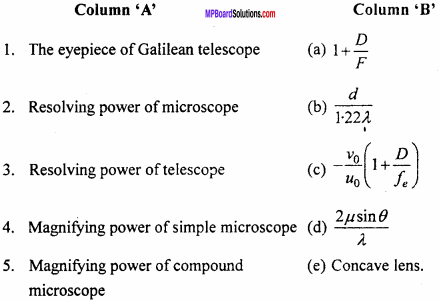1. (e)
2. (d)
3. (b)
4. (a)
5. (c)

II.1. (c)
2. (e)
3. (d)
4. (b)
5. (a)

Question 4.
Write the answer in one word / sentence:

1. If a ray of light incident normally on a mirror, then what will be angle of reflection?
2. From which spherical mirror we get magnified image?
3. From which spherical mirror we get always a virtual and smaller than object?
4. Write the relation between radius of curvature and focal length for a spherical mirror?
5. Conjugate foci is true for which type of images?
6. When a spherical mirror is immersed in water, what changes we get in its focal length?
7. What will be the magnitude of magnification of a convex mirror for its real image and virtual image?

1. Zero
2. Concave mirror
3. Convex mirror
4. f = $$\frac {R}{2}$$.
5. For real images
6. There is no changes in focal length
7. For real image it is negative and for virtual image it is positive.Question 4.
Write the answer in one word / sentence :

1. If a ray of light incident normally on a mirror, then what will be angle of reflection?
2. From which spherical mirror we get magnified image?
3. From which spherical mirror we get always a virtual and smaller than object?
4. Write the relation between radius of curvature and focal length for a spherical mirror?
5. Conjugate foci is true for which type of images?
6. When a spherical mirror is immersed in water, what changes we get in its focal length?
7. What will be the magnitude of magnification of a convex mirror for its real image and virtual image?

1. Zero
2. Concave mirror
3. Convex mirror
4. f = $$\frac {R}{2}$$
5. For real images
6. There is no changes in focal length
7. For real image it is negative and for virtual image it is positive.

Ray Optics and Optical Instruments Very Short Answer Type Questions

Question 1.
Which property remain unchanged in the phenomenon of refractions of light?
Frequency.

Question 2.
Which property get changed in the phenomenon of refraction of light?
Velocity, wavelength and amplitudes.

Question 3.
Write one characteristics of image formed by convex mirror.
Image is formed behind the mirror.Question 4.
If a water tank is seen inclinedly what will its apparent depth will be seen?
Its apparent depth will decrease when seen inclinedly.

Question 5.
What are the conditions for total internal reflection?

1. Angle of incidence should be more than critical angles.
2. Light rays must enter from denser medium to rarer medium.

Question 6.
On which phenomenon optical fibre is depend?
Total internal reflection.

Question 7.
When the value of relative refractive index is less than one and more than one?
Refractive index of rarer medium with respect to denser medium is less than one and refractive index of denser medium with respect to rarer medium is more than one.

Question 8.
If a concave mirror and convex lens is dipped in water, what will be the changes in their focal length?
The focal length of concave mirror will remain unchanged and that of convex lens will increase.

Question 9.
Can a lens be used in that medium from which it is made of?
No.

Question 10.
If an object is kept at focus of a concave lens where its image will formed?
Image will be formed between focus and optical centre of the lens.

Question 11.
The power of which lens be positive?
Convex lens.

Question 12.
The power of which lens is negative?
Concave lens.Question 13.
The power of a lens is more in water or air?
Air.

Question 14.
What is focal length and power of a rectangular slab of glass?
P = $$\frac {1}{f}$$ = $$\frac {1}{∞}$$ = 0. Power is zero.

Question 15.
Write the unit of Dispersive power.
It has no unit.

Question 16.
Write the formula for Rayleigh’s law.
I ∝$$\frac { 1 }{ { \lambda }^{ 2 } }$$

Question 17.
Why sky is seen blue?
Sky is seen blue due to scattering of light.

Question 18.
Write the formula for angular dispersion.
θ = A(µv – µr)
or θ = A(δv – δr).

Question 19.
When the light rays is parallel to the base of prism?
At angle of minimum deviation.

Question 20.
Write the relation between angular dispersion and dispersive power.
ω = $$\frac {θ}{δ}$$ = θ = ω x δ.

Question 21.
Which microscope is used as reading lens?
Simple microscope.

Question 22.
How resolving power of microscope depends on wavelength of light?
When wavelength of light decreases, its resolving power increases.

Question 23.
Write the formula of magnification for terrestrial telescope.
m = f1 – fe.

Question 24.
The length of which telescope is more among reflective or refractive telescope.
The length of refractive telescope is more.

Question 25.
How image is turned of simple microscope?
Erect and larger than object.Question 26.
How final image is formed for compound microscope?
Virtual, inverted and larger than object.

Question 27.
If the focal length of eye lens of a microscope is decreased then what will be the effect in its magnifications?
Its magnification will increase.

Question 28.
If the focal length of objective lens of microscope and telescope is increased, then what will be the effect in their magnifications?
Magnifications of microscope will decrease and that of telescope will get increased.

Ray Optics and Optical Instruments Short Answer Type Questions

Question 1.
What is mirror? What are the types of it?
The highly polished reflective surface is called mirror, it is of three types :

1. Plane mirror
2. Concave mirror
3. Convex mirror.

Question 2.
Write the definition of convex mirror and concave mirror.
1. Convex mirror:
If the internal surface of a spherical mirror is polished and reflection of light occur from external surface, it is called convex mirrors.

2. Concave mirror:
If the external surface of a spherical mirror is polished and reflection of light occur from internal surface, it is called concave mirror.

Question 3.
Write the formula for focal length of spherical mirror and explain it.
$$\frac {1}{f}$$ = $$\frac {1}{v}$$ + $$\frac {1}{u}$$ where f is focal length, v is image distance and u is object distance for a spherical mirror.

Question 4.
Define magnification of a spherical mirror.
The ratio of size of image to size of object is known as magnification.
m = $$\frac {h’}{h}$$ = $$\frac {v}{u}$$

Question 5.
Concave mirror is used for shaving, why?
When the object is within the focal length of the concave mirror then virtual, erect and magnified image is formed. This is the reason that concave mirror is used for shaving.

Question 6.
If a glass slab is kept in alphabet of different colour. Will all the alphabet be seen to be raise uniformly?
No, red colour alphabet will be seen to be raised more as its refractive index is less.

Question 7.
What is the cause of brilliance of diamond?
Diamond shines due to total internal reflection. Refractive index of diamond is 2.42 and critical angle is 24.41°, which is very less. Diamond is cut in such a way that light is incident on its faces at an angle more than 24.41°. The incident light undergoes total internal reflection repeatedly and hence gets collected inside, but it comes out only through few faces. Hence diamond sparkles when seen in the direction of emergent light.Question 8.
What will be the effect on the focal length of a concave mirror and convex lens when they are immersed in water?
The focal length of concave mirror will remain same but the focal length of convex lens will be increased.

Question 9.
Define refractive Index.
When the light rays enter from one conjugate medium to another conjugate medium, the ratio of sine of angle of incidence to sine of angle of refraction is known as refractive index of medium.

Question 10.
Write the reason for twinkling of stars.
As we go on higher and higher altitude the density of air goes on decreasing and hence the refractive index. Also, the atmosphere consists of several moving masses of air and the temperature is also changing, because of all these reasons the rays coming from the stars continuously changing the path.

Therefore, the quantity of rays entering the eye of an observer, changes. When these rays enter the eye the star appears brighter and so. Thus, the stars appear twinkling. The moon is very near to the surface of earth. The light coming out of the moon does not undergo much changes when it crosses the earth’s atmosphere. So, moon does not appear twinkling.

Question 11.
Write any two conditions for total internal reflection.

1. The ray of light must travel from denser medium to rarer medium.
2. The angle of incidence (i.e., critical angle) for which angle of refraction should be 90°.

Question 12.
Write the formula for refraction for a lens.
$$\frac {1}{f}$$ = (µ – 1)($$\frac { 1 }{ { R }_{ 1 } } -\frac { 1 }{ { R }_{ 2 } }$$)

Question 13.
Define power of a lens.
The reciprocal of focal length of a lens in metre is called its power.
p = $$\frac {1}{f}$$(m)

Question 14.
A concave lens and a convex lens of same focal length is kept in contact. What will be the focal length and power of the combination?
$$\frac {1}{F}$$ = $$\frac { 1 }{ { f }_{ 1 } } +\frac { 1 }{ { f }_{ 2 } }$$
f1 = – f and f2 = f
∴ $$\frac {1}{F}$$ = –$$\frac {1}{f}$$+$$\frac {1}{f}$$ = 0
or F = 0
and power p = $$\frac {100}{f}$$ = $$\frac {100}{∞}$$

Question 15.
What do you mean by magnification? Write its formula.
The ratio of sine of image to sine of object is defined as magnifications, its formula is
m = $$\frac {I}{O}$$ = $$\frac {v}{u}$$

Question 16.
Draw ray diagram of image formation when an object is placed at focus of a convex lens.
The image formed at infinity. Image is real and inverted.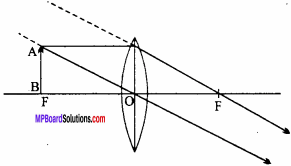Question 17.
A glass prism causes dispersion while a glass plate does not. Why?
When white light passes through a prism, it splits up into its constituent colours because refractive index of glass is different for different colours. The angular dispersion is (µV – µR) A and angular deviation is (µ – 1) A. The refracting faces of glass plate are parallel, so, A = 0. Hence, there is neither dispersion nor deviation. All the emergent rays are parallel to incident ray.

Question 18.
Define dispersive power.
The ratio of the angular dispersion between the violet and red emergent rays and the deviation suffered by the mean ray (yellow colour) is called dispersive power (ω) of the material of the prism.Question 19.
Define angular dispersion. On what factors it depends and how?
When light rays get dispersed into constituent colours, angle between any two colours (in general violet and red) is called angular dispersion.
If δV is the deviation for violet light and SR for the red light, then angular dispersion (θ) is,
θ = δV – δR.Question 20.
Danger signals are always red, why?
When light falls on the signal, all colours are scattered much more than that of red colour. So it suffers least scattering and reachs directly to the eye of the observer. Hence it can be seen from far – off distances.

Question 21.
Sun appears red during the sunrise and sunset, why?
Sunlight has to travel through the longer distances at the time of sunset and sunrise, meanwhile almost all the coloured light waves get scattered except red as its wavelength is more, it is unable to scatter due to atmospheric molecules. Therefore, only red light rays reach our eyes and sun appears red.

Question 22.
What do you mean by dispersion and spectrum?
When white light passes through the prism, it gets splitted into its constituent colours. This process is called dispersion. Colours in group is called spectrum. Order of colours in spectrum is violet, indigo, blue, green, yellow, orange, red.Question 23.
Why does the light rays disperse through prism?
White light consists of continuous range of wavelength. Refractive index of prism material is different for different colours and is given by Cauchy relation :
µ = a + $$\frac { b }{ { \lambda }^{ 2 } } +\frac { c }{ { \lambda }^{ 2 } }$$
Where a. b and c are constants. For small angle of prism, the angle of deviation is given by
δ = (µ – 1)A ⇒ δ ∝µ
Now,
λred > λviolet
∴ µred < µviolet
∴ δred < δviolet
i.e., the red colour is deviated less than the violet colour. Other colours are deviated by intermediate angles. So, different colours of white light get dispersed on refraction through prism.

Question 24.
What do you mean by angular dispersion? Derive an expression for it. On “what factors does it depends?
The angular dispersion is defined as difference between angle of deviations for any two colours. If angle of deviations for red and violet colours are δr and δv respectively then,
Angular dispersion θ = δv – δr
∴ δv = A(µv – 1), δr = A (µr – 1)
∴ θ = A(µv – 1) – A(µr – 1)
or = θ = A(µv – µr)
This is the required expression for angular dispersion.
θ depends on :

1. Angle of prism (A) : With increase in A, θ increases.
2. Material of prism : With increase in ‘µ’ θ also increases.

Question 25.
If the aperture of the objective lens of telescope is increased, then what would be the effect on :

1. Magnifying
2. Resolving power

1. The magnifying power remains unchanged.

2. Resolving power is increased as
Resolving power = $$\frac {a}{1.22λ}$$
Where, a = aperture.

Question 26.
How the magnifying power of an astronomical telescope is increased?
The magnifying power of an astronomical telescope is given by :
m = – $$\frac { { f }_{ 0 } }{ { f }_{ e } }$$
Hence, for greater magnifying power of the value f0, i.e., focal length of objective lens should be large and fe, i. e. focal length of eye lens should be small.

Question 27.
If the telescope is inverted, then the size of the image becomes very small, but it does not happen in microscope. Why?
The magnifying power of a telescope is m = – $$\frac { { f }_{ 0 } }{ { f }_{ e } }$$. If the telescope is inverted, then the magnification will become m = – $$\frac { { f }_{ 0 } }{ { f }_{ e } }$$. As fe << f0 hence, the size of the image will be very less.

In compound microscope the magnification is m = – $$\frac { { v }_{ 0 } }{ { u }_{ 0 } } \frac { D }{ { f }_{ e } }$$ The value of u0 is little more than f0. Hence, the formula for magnification can be written as m = – $$\frac { { v }_{ 0 } }{ { u }_{ e } } \frac { D }{ { f }_{ e } }$$ reversing the microscope, the angle subtended by v0 will be the same. So, the magnification remains unchanged.

Question 28.
The moon is several times smaller than sun. Even then at the time of eclipse, the entire sun is covered by moon. How?
The distance of sun from the earth is 1000 times much larger than the distance of moon from earth. During solar eclipse, the moon comes in between sun and earth. But the sun and moon subtend equal angle at our eyes. Hence, the moon covers the sun completely.

Question 29.
What do you mean by the resolving power of a microscope?
Resolving power:
The resolving power of a microscope is determined by the least distance between two point objects which can be distinguished by the microscope.
Resolving power = $$\frac {2µsinθ }{λ}$$ Where, µ is refractive index of the medium between the object and objective, λ is the wavelength of light used to illuminate the object, θ is the semivertical angle of the cone of light from the point object.

Question 30.
What do you mean by the resolving power of a telescope?
Resolving power:
The resolving power of a telescope is defined as the reciprocal of the smallest angular separation between two distant objects whose images are separated in the telescope.
i. e., Resolving power = $$\frac {a }{1.22λ}$$
Where, X is wavelength of light and a is the diameter of objective lens.Question 31.
In compound microscope, why the focal length of objective should be less than that of eyepiece?
Or
Why the focal length of the objective of a compound microscope should be small?
The magnifying power of a compound microscope is given by :
m = – $$\frac { { v }_{ 0 } }{ { u }_{ 0 } }$$ (1 + $$\frac { D }{ { f }_{ e } }$$)
For greater magnifying power u0 should be smaller, but the object should be placed out of the focus of the objective lens. Therefore, the focal length of the objective lens should be small.

Question 32.
What is astronomical telescope?
The telescope by which we can observe the objects at space is known as astronomical telescope.

Question 33.
Is it possible that, when a microscope is inverted, it becomes telescope? Give reason.
No, because of the difference of focal lengths of objective and eyepiece in microscope is less while in telescope it is greater.

Question 34.
Microscope and telescope must have high magnifying power and resolving power. Why?
When magnifying power is high The object is seen big and clear whereas for low resolving power. There is no clearity of objects.

Question 35.
Why the aperture of photographic lens is less?
When the aperture of photographic lens is less, the depth of focus of it increases.

Question 36.
What is reflection? Write the laws of reflection.
Reflection:
The phenomenon of bouncing back of the part of light in the same medium when light is incident on the polished surface (minor surface) is called reflection of tight.Laws of reflection :

1. Angle of incidence is always equal to the angle of reflection (i.e., i = r).
2. The incident ray, reflected ray and normal at the point of incidence all lie on the same plane.

Question 37.
Show the position, nature and size of image when an object is placed between pole and focus infront of concave mirror.
Let a ray parallel to principal axis incident from point A on the mirror and reflected through F. Another ray is incident normally and reflects back tracing the same path. These rays after reflection appears to come from point A’. Hence A ‘B’ be the virtual image of object AB.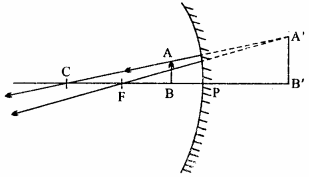Thus image is virtual, erect and larger in size than object.

Question 38.
What are conjugate foci?
The pair of points on the principal axis of a spherical mirror, such that the object placed at one of the point forms the image on the another point, are called conjugate foci. It is applicable only for real image.

Question 39.
What is parallax?
When two objects are seen on a straight line, they seem to be coincident. If the objects are at different places and the eye is moved sideways then there is a relative displacement between them. Thus, when two objects are seen in a straight line and the eye is moved side ways, then relative displacement is called parallax.Question 40.
What type of mirror is used in vehicle to observe the traffic at the back?
Convex mirror, as the image formed by a convex mirror is virtual, erect and smaller, therefore wider field of view of the traffic is given by the convex mirror.

Question 41.
What are the factor upon which refractive index of the medium depends?

1. On the pair of medium : Refractive index of denser medium with respect to rarer medium is always greater than 1 but refractive index of rarer medium with respect to denser medium is less than 1.
2. Density of the medium : If optical density of any medium is more than its refractive index will also be more.
3. Wavelength of light : If the wavelength is less more is the refractive index and more the wavelength less is the refractive index.
4. Temperature of medium : On increasing temperature, it becomes optically rarer hence refractive index decreases.

Question 42.
What is principle of reversibility? Explain.
Principle of reversibility:
If the path of a r’of light after number of reflections or refractions, is reversed, then the ray retraces the same path in opposite direction i.e., it will follow the same path by which it came.In fig. PQ is a refractive surface (air – glass interface) made of glass.
AO is incident ray and OB is refracted ray. If the path of ray is reversed, then it return back in OA direction.
Obviously, aµg= $$\frac {sin i}{sin r}$$ … (1)
Where, i is angle of incidence and r is angle of refraction.
Now, if light ray refracts from glass to air, then
a = $$\frac {sin r}{sin i}$$ … (2)
From equation. (1) and (2),
g × gµa = $$\frac {sin i}{sin r}$$ × $$\frac {sin r}{sin i}$$
or aµg × gµa = 1
This is principle of reversibility.

Question 43.
Refractive index of diamond is much greater than normal glass. Why?
Refractive index of diamond (µ = 2.42) is much greater than normal glass (µ = 1.5) so the critical angle of diamond is approximately 24°. Due to this for diamond. The light rays which incidences between 24° to 90° suffer’s total internal reflection. Number of turns due to which the brilliance of diamond increases.

Question 44.
What do you understand by refraction of light? Write its law.
The phenomenon of change in the path of light as it passes obliquely from one transparent medium to another is called refraction of light.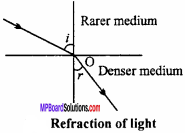Laws :
1. The incident ray, refracted ray and normal to the surface at the point of incidence all lie on the same plane.

2. For a monochromatic light the ratio of sine of the angle of incident to the sine of angle of refraction is constant for given pair of medium i.e., $$\frac {sin i}{sin r}$$ = µ
Where µ is known as refractive index of the medium.

Question 45.
Define the following for lens :

1. Optical centre
2. Conjugate foci
3. First and second principal focus.

1. Optical centre:
If the emergent ray from the lens is parallel to incident ray, then the point where the refracted ray intersect principal axis is called optical centre.2. Conjugate foci:
It is a pair of points on the principal axis such that when an object is placed at one point its image forms at the another and when the object is placed at the second point the image is formed at the first point. Such pair of points are called conjugate foci.3. First principal focus F1:
It is a point on principal axis of a lens such that the rays of light starting from it (convex lens) or appearing to meet at it (concave lens), after refraction from the lens become parallel to principal axis.

Second principal focus F2:
It is the point on the principal axis of lens such that the rays of light incident parallel to principal axis after refraction from the lens pass through this point (convex lens) or appear to diverge from this point (concave lens).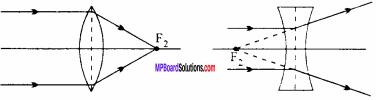Question 46.
When an object is kept in between optical centre and focus in front of convex lens draw a ray diagram of image formation.Question 47.
Write Cartesian sign convention for a lens. According to it which lens will have positive and negative focal length?

1. The incident ray is taken from left to right for drawing ray diagram.
2. All the distance measured in the direction of incident ray is taken as positive and opposite to it is taken as negative.
3. Height measured perpendicular to principal axis taken as positive and downwards taken as negative.

The focal length of convex lens is taken as positive whereas for concave lens it is negatives.

Question 48.
What do you mean by linear magnification? For a convex lens show that:
$$\frac {I}{O}$$ = $$\frac {v}{u}$$, where symbols have their usual meanings.
The ratio of the size of the image to that of the size of the object measured perpendicular to principal axis is called linear magnification.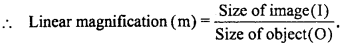Expression for magnification :This is the required formula.

Question 49.
On which factor the focal length of any lens depends?
1. Material of the lens:
$$\frac {1}{f}$$ = (µ – 1)($$\frac { 1 }{ { R }_{ 1 } } -\frac { 1 }{ { R }_{ 2 } }$$) or $$\frac {1}{f}$$ ∝ µ or f ∝$$\frac {1}{µ}$$ i.e., focal length will be greater for smaller refractive index.

2. Colour of light or wavelength:
The focal length for violet colour is lesser than that of red colour for any lens.

For thick lens (R is more) focal length is less whereas for thin lens (R is less) focal length is more.

Question 50.
Explain the formation of image in case of concave lens.
Let AB be an object kept perpendicular to principal axis. An incident ray parallel to principal axis appear to passing through principal focus (F). Second rays which passes through optical centre O after refraction moves in straight line. When both refracted ray are traced beyond they meet at point A’ where a vertical image is formed. Hence A’ B’ is the final image of the object.Question 51.
When a lens is dipped in liquid its focal length increases, why?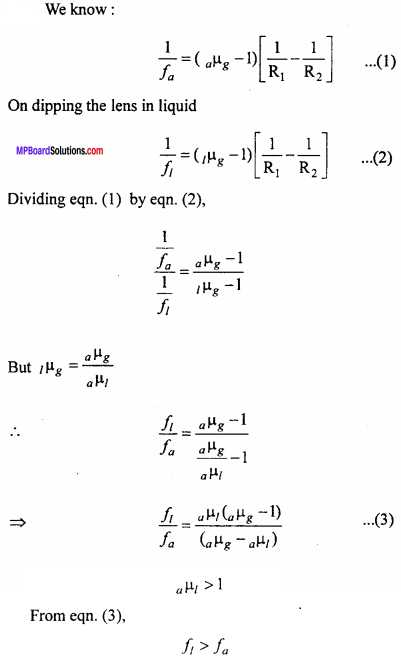When a lens is dipped in liquid its focal length increases.

Question 52.
Why does sky appear blue?
According to the Rayleigh, light rays with smaller wavelengths are scattered more and we know that blue colour light has smaller wavelength than the red light. There fore, when light falls on atmospheric molecules, blue light waves scatter more and spread into the sky. That is why, sky appears blue. As moon has no atmosphere hence there will be no scattering of light. Hence, sky will appear dark.

Question 53.
What is scattering of light? Explain scattering with the help of theory of electromagnetic waves.
Scattering of light:
When light falls on particles of size smaller than wavelengths of light much of the light is picked up by particle and given out in some other direction. This is known as the scattering of light.

Rayleigh’s law:
According to Rayleigh, the intensity of scattered light is inversely proportional to the fourth power of wavelength of light.
i.e I ∝$$\frac { 1 }{ { \lambda }^{ 4 } }$$ As the wavelength ofBlue light is less than that of red light, therefore blue light is scattered more than red light.Question 54.
How refraction of light occur in prism? Explain.
As shown in fig., let ABC is a prism, AB and AC are its refracting faces. Suppose a light ray PQ is being incident at point Q of the surface AB. MO is normal drawn at point Q.As at point Q the light ray is entering from air (rarer medium) to glass (denser medium), so it bends towards normal following the path QR and falls at point R of the surface AC, NO is normal at point R. At point R the refraction of light ray takes place from glass (denser medium) to air (rarer medium), so it goes away from normal and emerges out in RS direction. Obviously PQ be the incident ray, QR be the refracted ray and RS be the emergent ray.

∠PQM = angle of incidence (i), ∠RQO = angle of refraction (r) and SRN = angle of emergence (e). So, it is clear that when light ray passes through prism n gets deviated from us path. The deviation is measured by angle between direction of incident ray and emergent ray. The angle between incident ray and emergent ray is called angle of deviation. It is represented by S. In fig, UTS is an angle of deviation.

Question 55.
What are the factors on which angle of minimum deviation depends?
1. Angle of prism:
It is clear from eqn. (6) that δm ∝A , so if the value of angle of prism is greater than the value of minimum angle of deviation will also be greater.

2. Refractive index of material of prism:
δm ∝ (µ – 1), so greater the value of refractive index of material of prism greater will be the value of angle of minimum deviation.

3. Colour of light:
The value of angle of minimum deviation also depends upon colour of light. Refractive index of prism is greater of violet colour and is less for red colour. So, violet colour is deviated most and minimum deviation will be for red.

Question 56.
What is angle of minimum deviation. Draw its graphs.
It has been found by the experiments that the value of angle of deviation depends on the angle of incidence. As the value of angle of incidence is increased, the value of angle of deviation decreases. For a particular value of angle of incidence the angle of deviation becomes minimum, further if the value of angle of incidence is increased, then the value of angle of deviation increases.

The minimum value of angle of deviation is called angle of minimum deviation. It is denoted by δm. In this position the prism is said to be under the position of minimumQuestion 57.
Write similarities between Astronomical telescope and Galilean telescope.
Similarities:
Astronomical telescope :

• The focal length of objective lens is greater than of eye lens.
• When final image is formed at infinity m = $$\frac { { f }_{ 0 } }{ { f }_{ e } }$$
• The aperture of objective is greater than that of eye lens.

Galilean telescope

• The focal length of objective lens of this telescope is also greater than that of eye lens.
• When final image is formed at infinity m = $$\frac { { f }_{ 0 } }{ { f }_{ e } }$$
• The aperture of objective is greater than that of eye lens.

Question 58.
Write dissimilarities between Astronomical telescope and Galilean telescope.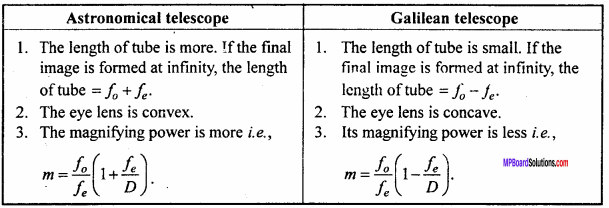Question 59.
Differentiate between magnifying power and resolving power of the telescope.
Difference between magnifying power and resolving power:
Magnifying power:

• It is defined by the ratio of visual angle of final image to visual angle of object.
• For greater magnifying power the focal length of objective should be greater and that of eye piece should be small.

Resolving power:

• It is defined as the reciprocal of the smallest angular separation between two distant objects whose images are separated in the telescope.
• For greater resolving power the diameter of objective should be greater.

Ray Optics and Optical Instruments Long Answer Type Questions

Question 1.
Establish the relation between focal length and radius of curvature for spherical mirror.
Or
Prove for spherical mirror:
f = $$\frac {R}{2}$$
Establish the relation between focal length and radius of curvature concave mirror.
Let MPN be a concave mirror and its pole is P, focus F, centre of curvature is C and PC is principal axis.
Now, a ray AB is incident on the mirror parallel to principal axis. Join CB, which will be the normal to the mirror.
Now, by laws of reflection,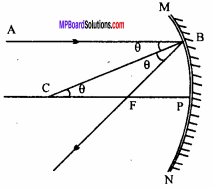Incident ∠ABC = Reflected ∠CBF = θ (let)
AB ∥ PC and BC is a transversal line
∠ABC = ∠PCB = θ, (alternate angles)
∠CBF = ∠PCB = θ
∴ In ∆BCF, the opposite sides will be equal.
∴ FC = BF
As the aperture of the mirror is small.
∴ BF = PF (approx)
∴ FC = PF
or PC = PF
or R = 2f
or f = $$\frac {R}{2}$$Question 2.
Define focal length of a spherical mirror. Find out the relation between u, v and f for spherical mirror.
Or
What are conjugate foci? For any spherical mirror prove that:
$$\frac {1}{f}$$ = $$\frac {1}{u}$$ + $$\frac {1}{v}$$

Or
Establish the relation amongst u, v and f for concave mirror.
Or
What is mirror formula? Derive mirror formula for spherical mirror.
Focal length:
The distance between the principal focus and pole of mirror is called focal length of spherical mirror.

Conjugate foci:
The pair of points on the principal axis of a spherical mirror, such that the object placed at one of the point forms the image on the another point, are called conjugate foci. It is applicable only for real image.
Let MPN is a concave mirror, P is pole, F is a focus and C is its radius of curvature.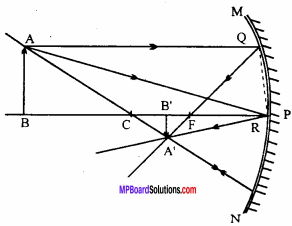If A’B’ is an image of any object AB placed on principal axis, before the mirror.
Now, join AP and AP. AP is an incident ray and PA’ is a reflected ray at point P.
Draw a normal on principal axis from Q.
Now, ∆ A’B’F and ∆ QRF are similar.Question 3.
Prove that aµw x wµg x gµa = 1
When a ray of light passes through several parallel media and if the first and last medium is same, then the incident and emergent beam will be parallel to each other but they will be laterally displaced.
Referring to Fig., let AB be an incident beam falling on the air – water interface at an angle of incidence i. It undergoes refraction and bends towards the normal as BC with angle of refraction as r1.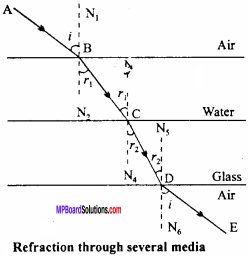By Snell’s law,
aµw = $$\frac { sini }{ sin{ r }_{ 1 } }$$ … (1)
At C, the beam BC undergoes refraction at the water – glass interface and moves as CD.
∠N2BC = ∠BCN3 = r1 (alternate angles)
So, at the water – glass interface, r1 is the incident beam and r2 is the refracted beam. Hence, by Snell’s law,
wµg = $$\frac { sin{ r }_{ 1 } }{ sin{ r }_{ 2 } }$$ … (2)
At D, the beam CD strikes the glass-air interface. As the beam moves from denser to rarer medium, so it will bend away from the normal. But all the interfaces are parallel to each other and the first and last medium are the same, hence the angle of emergence is equal to angle of incidence.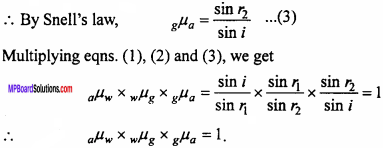Question 4.
Establish relation between real and apparent depth, tns. Let an object P is placed at the bottom of a water tank (denser medium). An observer is observing that object. A light ray PQ coming from object incident at Q and get refracted. Another ray of light falls normally on the water air interface and refracts without deviation. These refracted rays appears to meet at point P’.
∴ ∠OPQ = ∠PQN = i (alternating angles)
∠OPQ = ∠MQR = r (corresponding angles)
Let PO = h(alternating angles)
and P’O = h'(corresponding angles)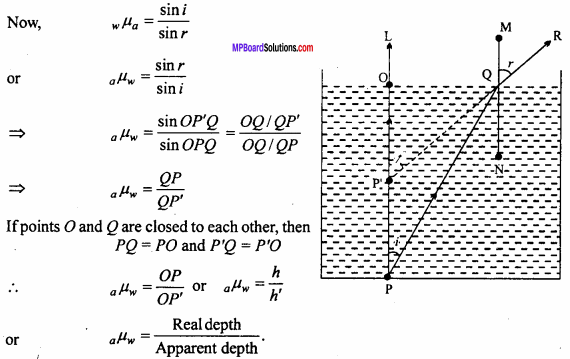Question 5.
What is total internal reflection? Write its conditions. Establish the relation refractive index of the medium and critical angle. On what factors does the critical angle depend?
Or
Establish the relation between critical angle and refractive index of medium.
Critical angle:
When ray of light travel from denser medium to rarer medium. It is the angle of incidence for which the angle of refraction is 90°.
Total internal reflection:
When light ray moves towards rarer medium from denser medium and if angle of incidence is greater than the critical angle, light ray cannot pass through the plane joining the two media and gets reflected back to the same medium. This phenomenon is called total internal reflection.Conditions:

1. Light ray must travel from the denser medium to rarer medium.
2. Angle of incidence should exceed the critical angle value.

Relation between critical angle and refractive index:
Let PQ is an incident ray travelling through denser medium to rarer medium and refracted along QR.
∴ Angle of incidence = ic
and angle of refraction = 90°
gµa = $$\frac { sin{ i }_{ c } }{ sin90° }$$ = sin ic
or gµa = $$\frac { 1 }{ { _{ a }{ \mu }_{ g } } }$$ = sin ic, (by principle of reversibility of light)
gµa = $$\frac { 1 }{ sin{ i }_{ c } }$$
Which is the required relation.

Examples:

1. Formation of mirage in desert
2. Brilliance of diamond.

Factors affecting the critical angle :
1. Colour of light ray used : The refractive index for violet ray is greater therefore critical angle will be least for violet colour (because c ∝$$\frac {1}{µ}$$).

2. The nature of pair of media:
The critical angle also depends upon the pair of media used. For glass-air pair its value is 42°. For diamond air pair its value is 24°.

3. Temperature:
With the increase of temperature the refractive index of the material of medium decreases therefore the critical angle increases.Question 6.
What are optical fibres? Write its construction and working method with diagram. Writfe any two uses of optical fibres.
Or
What afe optical fibres? Write its working principle. How does it transmit light signal? Write its main uses.
An optical fibre is a thin strand of highly pure glass or quartz core with outside coating of glass or slightly different chemical composition having different refractive index, as shown in fig.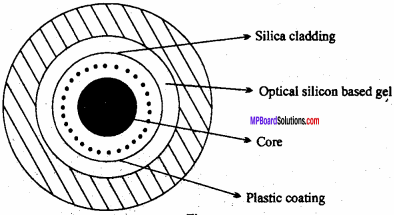The main parts of optical fibre are as follows :
1. Central core:
It is the innermost core of the fibre made of thin and fine quality transparent glass. The refractive index of material of core is about 1.7.It is a layer made of glass or plastic which surrounds the central core of optical fibre. Its refractive index is less than that of central core about µ = 1.5.

3. Plastic jacket:
The central core and cladding are enclosed in a protective jacket made of plastic for providing safety and strength to optical fibre.

Working:
When light falls on the interface separating the core and coating at an angle of incidence greater than the critical angle, then the phenomena of total internal reflection takes place because the refractive index of coating is less than the core. This phenomena take place continuously and light travels the entire length of the fibre even if it is curved or twisted.

Uses:

1. For transmission of light without any loss in its intensity.
2. In endoscopy, to examine the internal parts of body such as stomach and intestines.

Question 7.
What will be the effect on focal length and nature of a lens when dipped in liquid?
Media of both the sides of the lens : If the focal length of a lens in air is fa, thenand if the focal length of a lens in a liquid is f1, than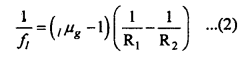Dividing equation (1) by equation (2), we get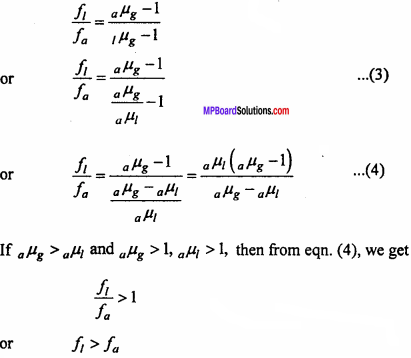Hence, in the liquid, focal length of lens increases.

Case I.
If the lens is dipped in a liquid whose refractive index is less than that of lens, i.e.,Hence, the nature of lens will remain the same.

Case II.
If the lens is dipped in a liquid whose refractive index is more than that of lens, i.e.,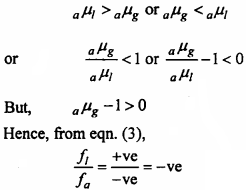So, the nature of the lens will change i.e., the lens which behaves as convex in air will behave as concave in liquid. This is the reason why an air bubble appears as concave lens in water.

Case III.
If the refractive index of lens is equal to that of liquid, then aµ1 = aµg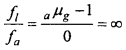Hence, the lens will behave as glass plate.

Question 8.
Prove for any spherical refracting surface :
$$\frac { \mu -1 }{ R }$$ = $$\frac { \mu }{ v } -\frac { 1 }{ u }$$
Where symbols have their usual meanings.
Or
Derive an expression $$\frac { \mu }{ v } -\frac { 1 }{ u }$$ = $$\frac { \mu -1 }{ R }$$ showing relation among p,u, v and R for aconcave spherical refracting surface.
Let APB is a cross – section of any spherical refracting surface. P is its pole and C is its centre of curvature. Left of the surface has air as a medium and in right, medium has refractive index µ.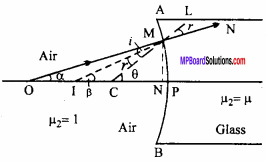Any point object is placed at point O on the principal axis of which a virtual image is formed at I due to refracting surface APB. According to the figure, angle of incidence,
∠OMC = i
Angle of refraction,
∠LMN = ∠IMC = r
Let ∠MOP= α, ∠MIP = β and ∠MCP = θ.
From Snell’s law,
µ = $$\frac {sin i}{sin r}$$
or µ = $$\frac {i}{r}$$, (as i and r are very small)
or i = µr … (1)
From ∆OMC, θ = i + a, (∵exterior angle = sum of opposite interior angles)
or i = θ – α … (2)
and in ∆ IMC, θ = r + β
or r = θ – β … (3)
From eqns. (2) and (3), putting the values of i and r in eqn. (1),
θ – α = µ(θ – β)
or µβ – α = (µ – 1)θ … (4)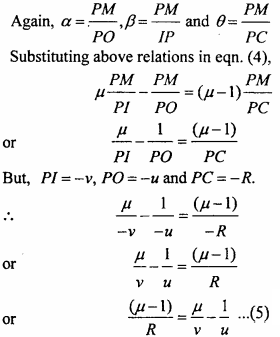This is the required formula.

Question 9.
Two lenses of focal lengths f1 and f2 are kept in contact, if the focal length of the combination is F, then prove that :
$$\frac {1}{F}$$ = $$\frac { 1 }{ { f }_{ 1 } } +\frac { 1 }{ { f }_{ 2 } }$$
Or
Establish the formula for the focal length of the combination of two thin lenses.
Or
Two thin convex lenses having focal lengths f1 and f2 respectively are kept in contact. Describe the method to determine the focal length of the system on the basis of following points:

1. Labelled ray diagram
2. Derivation of formula used
3. Two important precautions.

L1 and L2 are two convex lenses in contact with each other. P is the object pin whose final image is formed at Q after refracting through lenses L1 and L2. The image of P is formed at R due to lens L1 with optical centre O1 . The image of P at R acts as image for the lens L2 which forms its final image at Q.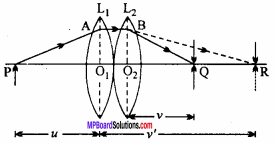The optical centre of L2 is O2 , u is the object distance of the pin P for lens L1 and v’ is the image distance for the lens L1 which acts as the object distance for the lens L2 forming the final image at Q at a distance v from L2.

Determination of formula:
Two lenses of focal length f1 and f2 are in contact with each other. The focal length of the combination is ‘f’ P is the object on the common principal axis of the two lenses. The image formed by L1 is at R. The image by L2 acts as object for the lens L2 which in turn forms the final image at Q.
∴ PO1= u, O1R = v’, O2Q = v
From the relation,
$$\frac {1}{f}$$ = $$\frac {1}{v’}$$ – $$\frac {1}{u}$$
For L1 of focal length f1,
$$\frac { 1 }{ { f }_{ 1 } }$$ = $$\frac {1}{v’}$$ – $$\frac {1}{u}$$ … (1)
For L2 of focal length f2,
$$\frac { 1 }{ { f }_{ 2 } }$$ = $$\frac {1}{v}$$ – $$\frac {1}{v’}$$ … (2)
Adding eqns. (1) and (2), we get
$$\frac { 1 }{ { f }_{ 1 } }$$+$$\frac { 1 }{ { f }_{ 2 } }$$ = $$\frac {1}{v}$$ – $$\frac {1}{u}$$ … (3)
If the focal length of the combination is F, then
$$\frac {1}{F}$$ = $$\frac {1}{v}$$ – $$\frac {1}{u}$$ … (4)
From eqns. (3) and (4),By knowing u and v, the focal length of combination of lenses can be determined.

Precautions:

1. The tips of the pins and optical centres of the lenses must be in one line and also this line must be parallel to the optical bench.
2. The eye should be placed nearly 25 cm away while removing the parallax.
3. The lenses must be neat and clean.

Question 10.
Draw the ray diagram of refraction through a lens and derive the refraction formula.
Or
prove for a lens : $$\frac {1}{f}$$ = (µ – 1)($$\frac { 1 }{ { R }_{ 1 } } -\frac { 1 }{ { R }_{ 2 } }$$)
Or
Where, the symbols have their usual meanings.
Or
What is lens maker formula? Derive it for a convex lens.
Or
Derive refraction formula for a thin lens.
Let any lens is made up of two spherical surfaces AB and CD of radius of curvatures R1 and R2 . Any point object is placed on principal axis at O. Image of an object O is formed at 7, due to AB and this image acts as an object for the second surface CD and image is produced at I1. In this way object’s image through the lens is formed at 7.
Let distance of object O from the lens is u, Image I1 is at distance v1 , Image I is at distance v.
∴ Through the first refracting surface AB,$$\frac { (\mu -1) }{ { R }_{ 1 } }$$ = $$\frac { \mu }{ { v }_{ 1 } }$$ – $$\frac {1}{u}$$ … (1)Now, if u = ∞, then v = f
Substituting these values in equation (3), we haveWhich is refraction formula.

Question 11.
Draw ray diagram for refraction through a prism and establish a relation between refractive index of material of prism, angle of prism and angle of minimum deviation.
Let ABC be a prism, AB and AC are refracting surfaces. A is the angle of prism, PQ is incident ray, QR is refracted ray, RS is emergent ray, MO and NO are normals, i1, is angle of incidence, r1 is angle of refraction, e is angle of emergence, r2 is angle of incidence of AC.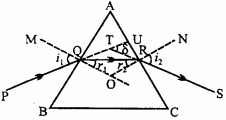Now, from figure,
Angle of deviation, δ = ∠UTS
or δ = ∠TQR + ∠TRQ,
(exterior angle is sum of interior opposite angles)
= (i1 – r1) + (i2 – r2)
In the position of minimum deviation,
i1 = i2 = i, (say)
and r1 = 2
∴ δm = (i – r) + (i – r) = 2i – 2r … (1)
∠AQO + ∠ARO= 180°, (each angle is equal to 90°)
∠A + ∠QOR = 180° … (2)
In ∠QOR,
∠QOR + r1 +r2 = 180° … (3)
From eqns. (2) and (3),
∠A + ∠QOR = ∠QOR + r1+ r2
or ∠A = r1 + r2
By condition of minimum deviation,
∠A = r + r = 2r or r = $$\frac {λ}{2}$$
Putting it in equation (1),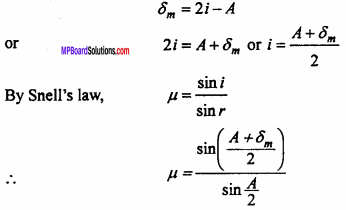This is the required formula

Question 12.
What is angle of minimum deviation? On what factor does it depend?
It has been found by die experiments that the value of angle of deviation depends on the angle of incidence. As the value of angle of incidence is increased, the value of angle of deviation decreases. For a particular value of angle of incidence die angle of deviation becomes minimum, further if the value of angle of incidence is increased, then the value of angle of deviation increases.

The minimum value of angle of deviation is called angle of minimum deviation. It is denoted by δm. In this position the prism is said to be under the position of minimum deviation.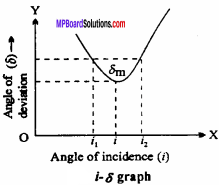Angle of deviation depends upon following factors :
1.Angle of prism:
It is clear that δm∝A , so if the value of angle of prism is greater then the value of minimum angle of deviation will also be greater.

2. Refractive index of material of prism:
δm ∝ (µ – 1), so greater the value of refractive index of material of prism greater will be the value of angle of minimum deviation.

3. Colour of light:
The value of angle of minimum deviation also depends upon colour of light. Refractive index of prism is greater of violet colour and is less for red colour.

So, violet colour is deviated most and minimum deviation will be for red.

Question 13.
What is rainbow? Explain the formation of primary and secondary rainbow with the help of ray diagram.
Sometimes, at the time of rain or after a shower, an observer standing with his back towards the sun looks a circular arc of spectrum colours in the sky, called rainbow.

Sometimes two rainbows are seen:

1. Primary rainbow and
2. Secondary rainbow.

In primary rainbow, red is seen outside and violet inside, while in secondary rainbow, the violet colour is at outside and red colour inside. In primary rainbow, the red colour emerges out at an angle of 42° and violet rays at an angle of 40°, hence the angular width of the rainbow is 2°. It is comparatively bright. In secondary rainbow, the violet rays emerges out at an angle of 55° and red rays at an angle of 52°. Hence, the angular width of the rainbow is 3°. It is comparatively less bright.

1. Primary rainbow : The primary rainbow is produced by two refractions and one total internal reflection of sun rays by the rain drops. It is shown in the Fig. (a).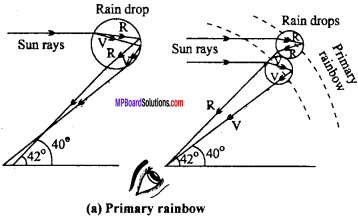2. Secondary rainbow: The secondary rainbow is formed by two refractions along with two internal reflections by the rain drops as shown in Fig. (b).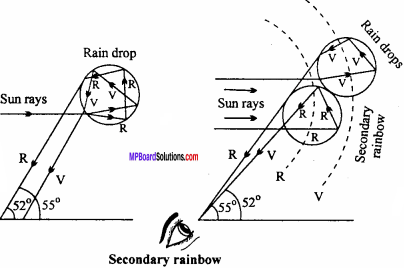Question 14.
What are differences between primary and secondary rainbow?
Differences between primary and secondary rainbow :
Primary rainbow:

• In this rainbow, red colour is outside and violet is inside.
• Its brightness is more.
• Its angular width is 2°.
• It lies below the secondary rainbow.
• It is formed by two refractions and one internal reflection.

Secondary rainbow

• In this rainbow, violet colour is outside and red is inside.
• It is less bright.
• Its angular width is 3°.
• It lies above the primary rainbow.
• It is formed by two refractions and two internal reflections.Question 15.
What is simple microscope? Draw the ray diagram and explain its principle. Write its two uses.
Or
Draw the ray diagram of simple microscope and obtain an expression for its magnifying power.
Or
Describe the working of simple microscope under following points:

1. Ray diagram for the formation of image when formed at D.
2. Derivation for the magnifying power when final image formed at D and at infinity.

Simple microscope is a device with the help of which magnified image of small objects are seen. It is a convex lens of less focal length.

Principle:
When an object is placed before a convex lens between its focus and optical centre then a virtual, erect and magnified image is formed.

Use:

2. To see the magnified image of small objects, example to see biological slides.

Ray diagram:
Ray diagram for a simple microscope is given below.

Expression for magnifying power :
The ratio of the visual angle of image to the visual angle of object, placed at the least distance of distinct vision, is called magnifying power.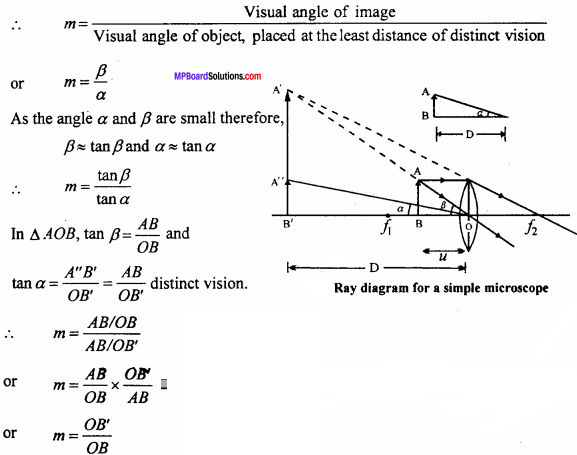Now OB’ = D, (least distance of distinct vision)
By sign convention OB’ = D(-ve) and OB = u(-ve)
∴ m = $$\frac {-D}{-u}$$
m = $$\frac {D}{u}$$
Where, u is the distance of object from the lens.
When the image is formed at the least distance of distinct vision :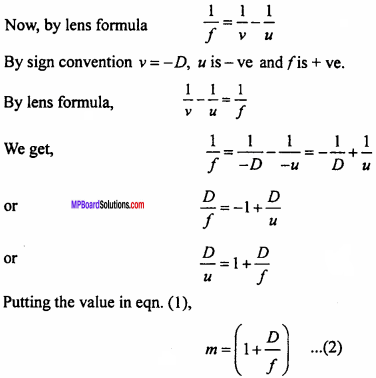When the image is formed at infinity: If the image is formed at infinity, then u = f.
∴ m = $$\frac {D}{u}$$ = $$\frac {D}{f}$$
For greater magnification the focal length should be small.

Question 16.
Describe compound microscope on following heads :

1. Ray diagram of formation of image.
2. Derivation of formula for magnifying power when the image is formed (a) At least distance of distinct vision, (b) At infinity, (c) Condition for greater magnification.

Or
Write the nature and kind of final image formed by compound microscope. How the magnifying power can be increased?
1. Ray diagram :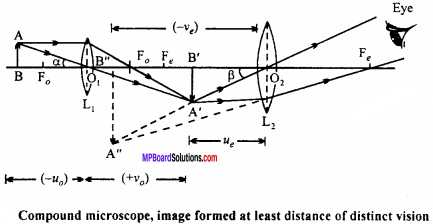2. Formula derivation for magnifying power:
The ratio of visual angle of image to the visual angle of object, placed at a distance of least distance of distinct vision is called magnifying power of compound microscope.If it is assumed that the object AB is kept at least distance of distinct vision D and the visual angle formed at eye is α, then
tan α = $$\frac {AB}{D}$$
The angle formed by final image A”B” is β.
∴ tan β = $$\frac {A”B”}{D}$$
If the angles α and β are very small, then tan α = α and tanβ = βIn Δ ABO1 and ΔA’B’O1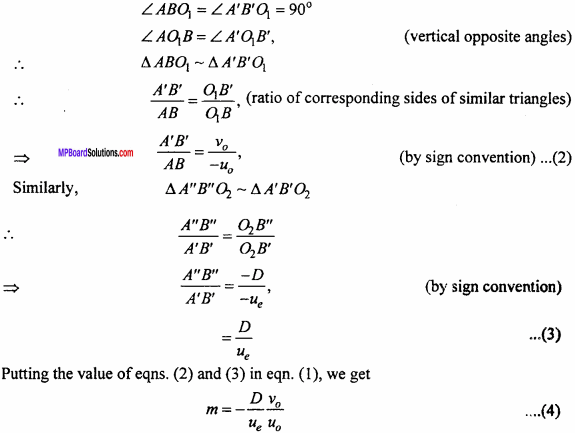(a) When the final image is formed at least distance of distinct vision :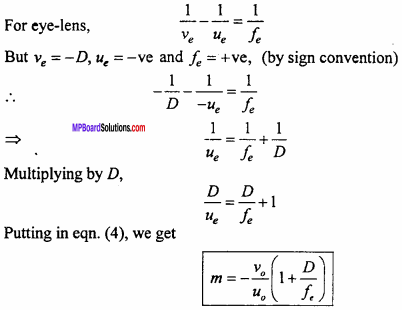This is the required formula, – ve sign shows that the image is inverted.

(b) When the final image is formed at infinity :
The eye – lens is so adjusted that the image A’B’ formed by the objective is on the focus of eyepiece, then ue = fe.
From equation (4),(c) Condition for better magnification :

1. u0 should be small, i.e., the object should be placed near the objective lens. But the object should be away from the focus, hence the focal length of the objective should be small.
2. fe should be small, i.e., focal length of eyepiece should be small.
3. v0 should be greater, i.e., the distance between objective lens and eyepiece should be greater.

Question 17.
Describe terrestrial telescope on following points :

1. Ray diagram
2. Calculation of magnifying power when the image is formed at (a) Least distance of distinct vision, (b) Infinity.

Terrestrial telescope:
These telescopes are used to observe the far object on the earth. In astronomical telescope image is formed inverted but in terrestrial telescope the image formed is erect. Therefore, these telescopes are used to observe the objects on earth.

1. Ray diagram :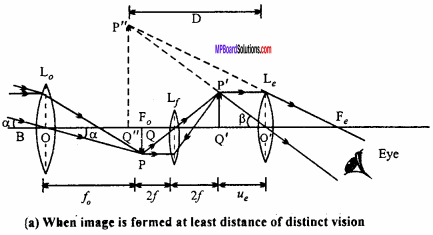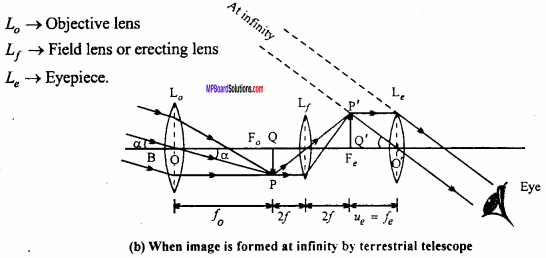2. Expression for magnifying power:
Magnification is defined as the ratio of the visual angle of final image to that of the visual angle of object. Visual angle of final image to that of the visual angle of object.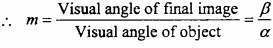From fig. (b),
Angle made by the final image P”Q” on eyepiece Le is
β = ∠P”O”Q” = ∠P’OQ’
Angle formed by object lens is
α = ∠P’OQ’
As the angles α and β are very small, hence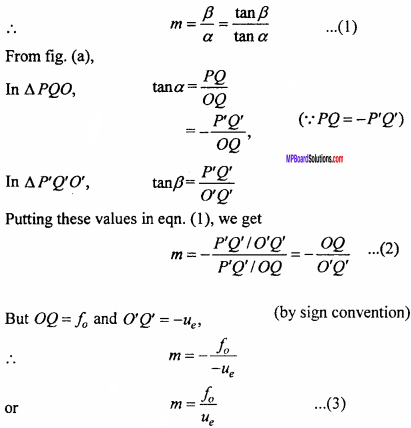(a) When the image is formed at least distance of distinct vision :
The image formed by the erecting lens lies within the focal length of eyepiece i.e., in between O’ and f, for eyepiece fe = ve and ve = -D.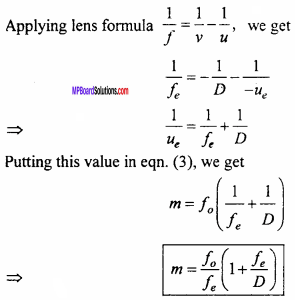(b) When the image is formed at infinity : In this case ue = fe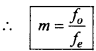Question 18.
Describe the astronomical telescope on following points :
(i) Ray diagram for the formation of image.
(ii) Formula derivation for magnifying power when the image is formed at (a) Least distance of distinct vision and (b) Infinity.
Length of telescope.
Or
Draw a labelled ray diagram of an astronomical telescope and going only formula for magnification. What are the conditions for maximum magnification?
1. Ray diagram for the formation of image:2. Formula derivation for magnifying power:
Magnification is defined as the ratio of the visual angle of final image to that of the object.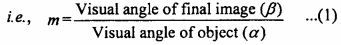From fig. (b),
Angle made by the final image P”Q” on eyepiece Le is
β = ∠P”O’Q’ = ∠P’OQ’
Angle formed by object on objective lens is
α = ∠P’OQ’
As the angles a and P are very small, hence(a) When the final image is formed at least distance of distinct vision:
The distance of eye – lens (Le) from final image P”Q” is O’Q” = ve = D(- ve). The object is P’Q’, situated at distance O’Q’ from eyepiece, i.e., ue = O’Q'(- ve).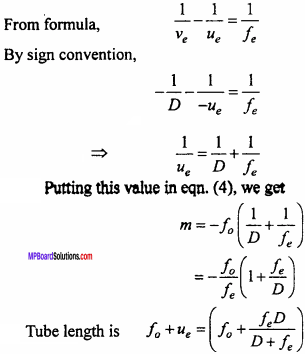(b) When the final image is formed at infinity:
The image p’Q’ formed by the objective lens (L0) lies on the first focus of eye – lens (Le) i.e., ue = fe
Putting in eqn. (iv), we get m = $$\frac { { f }_{ 0 } }{ { f }_{ e } }$$.

(iii) Tube length is f0 + fe.

Question 19.
Describe Galilean telescope under the following heads :

1. Ray diagram when final image is formed at least distance of distinct vision.
2. Expression for magnifying power when final image is formed at least distance of distinct vision D.

1. Ray diagram :2. Formula derivation for magnifying power:
Magnification is defined as the ratio of the visual angle of final image to that of the object.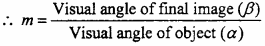Angle formed by final image β = ∠P’O2Q” = ∠P’O2Q’
Angle formed by object on objective lens α = ∠P’O1Q’
As angles and are very less, tanα ≈ α and tan β ≈ βIf the final image is formed at least distance of distinct vision : For eye – lens v = – D, u = ue and f = – fe, (By cartesian sign convention)In this case, the tube length is f0 – fe.

Ray Optics and Optical Instruments Numerical Questions

Question 1.
The focal length of a convex lens is 10 cm. If it is immersed into a liquid of refractive index 1.75, then find out its nature and focal length.
Solution:
Given, fa = 10 cm, aµ1 = 1.75, aµg = 1.5i.e., The lens behaves like a concave lens.

Question 2.
A lens forms an image of a needle placed at a distance of 45 cm from it, on a screen situated at distance 90 cm on other side of it. Name the kinds of lens. Calculate its focal length. If the size of needle is 5 cm, what will be the size of the image?
Solution:
Given, u = – 45 cm, v = 90 cm, size of object O = 5 cm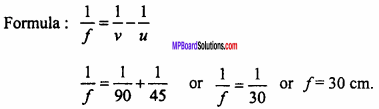∵ Focal length is positive therefore the lens would be a convex lens.
Again, magnification m = $$\frac {I}{O}$$ = $$\frac {v}{u}$$
or $$\frac {I}{5}$$ = $$\frac {90}{-45}$$
I = – 5 x 2 or I = – 10
(-) sign shows here that the image formed be inverted and real.Question 3.
Two thin lenses of power + 12D and – 2D respectively are placed in contact. Find the power focal length and nature of the combination.
Solution:
Given,
P1 = + 12D, P2 = – 2D
P = P1 + P2 = + 12D – 2D = 10D
But power = $$\frac {1}{f}$$
∴ f = $$\frac {1}{P}$$ = $$\frac {1}{10}$$m = 0.1 m
∵ Focal length is positive so the lens is of converging nature.

Question 4.
Velocity of light in glass (µ = 1.5) is 2 x 108m/s. Find out the velocity of light in air.
Solution:
Formula µ = $$\frac { { V }_{ a } }{ V_{ g } }$$ where aµg = 1.5, Vg =2 x 10 8m/s
Va =Vg x aµg = 1.5 x 2 x 108
or  Va = 3.0 x 108 m/s

Question 5.
The refractive index of glass and water are 3/2 and 4/3. Find the ratio of velocity of light in glass and water.
Solution:Question 6.
The refractive index of material of prism and its refracting angle are and 60° respectively. Find angle of incidence for the position of minimum deviation.
Solution: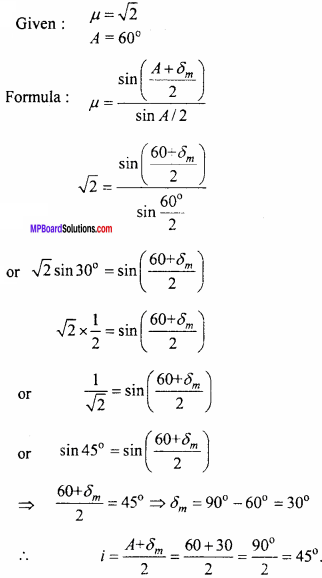Question 7.
Refractive index of water w.r.t. glass is $$\frac {9}{8}$$ if the velocity of light in water is 2.25 x 108m/s then find the velocity of light in glass.
Solution:Question 8.
The refractive indices for red and violet colour of a crown glass prism are 1.513 and 1.523 respectively. Find out dispersive power of crown glass prism.
Solution:Question 9.
The refractive angle of a prism is 60°. If angle of minimum deviation is 38° then obtain refractive index of material of prism, (sin 49° = 0.7547)
Solution: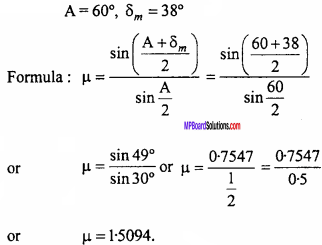Question 10.
If length of astronomical telescope is 80 cm and its magnification is 15, then find focal length of objective lens and eyepiece.
Solution:
Given : f0 + fe = 80 cm … (1)
m = – 15
Formula: m = – $$\frac { { f }_{ 0 } }{ { f }_{ e } }$$
– 15 = – $$\frac { { f }_{ 0 } }{ { f }_{ e } }$$
or f0 = 15 fe
put this value in equation (1),
15 fe + fe = 80
or 16 fe = 80
fe = 5cm
Now f0 + 5 = 80
or f0 = 75 cm.Question 11.
The magnifying power of an objective lens of a compound microscope is 8, if the magnifying power of the compound microscope is 32. Then calculate magnifying power of an eyepiece lens.
Solution:
Given: m0 = 8, m = 32, me = ?
Formula : m = m0 x me
32 = 8 x me
or me = $$\frac {32}{8}$$ = 4
or me = 4

Question 12.
Diameter of an objective lens of a telescopes is 01 m and the wavelength of light is 6000 A. What will be its resolving limit?